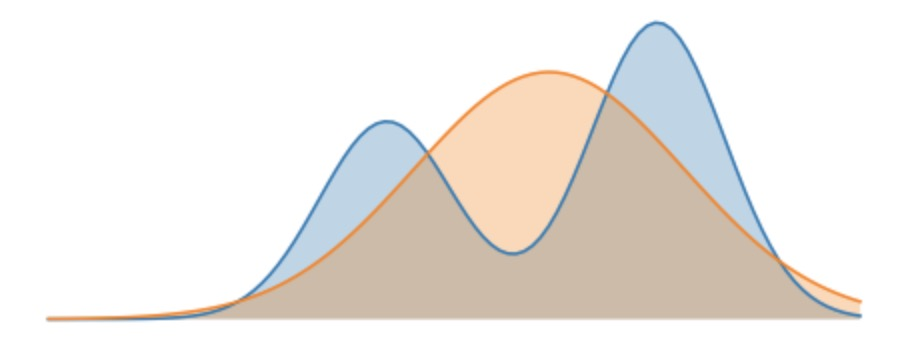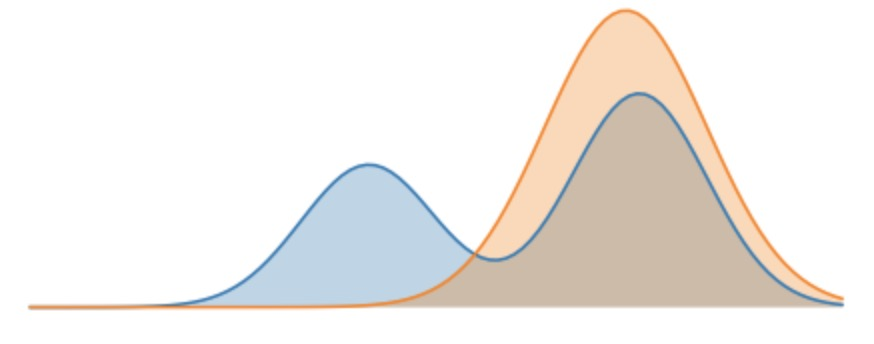KL Divergence and Maximum Likelihood

Posted by GwanSiu on June 12, 2019

1. KL Divergence

1.1 Definition of KL Divergence

The Kullback-Leibler (KL) divergence is a measure of how a probability distribution differs from another probability distribution. In Bayesian learning, data sample is assumed to be generated from the underlying distribution $P$, and we would like to estimate it with an approximated distribution $Q_{\theta}$ with parameter $\theta$. In this case, KL divergence can be applied to measure the distance between the approximated distribution $Q_{\theta}$ and the true distribution $P$.

In formal words, consider two probability distribution $P(X)$ and $Q_{\theta}(X)$. The KL divergence is defined as

KL divergence is not symmetric, i.e. $D_{KL}(Q_{\theta\Arrowvert P})\neq D_{KL}(P\Arrowvert Q_{\theta})$. Therefore, KL divergence is not a well-defined measure. If $P$ and $Q_{\theta}$ are exact the same, i.e. $P=Q_{\theta}$, then $D_{KL}(Q_{\theta\Arrowvert P})\neq D_{KL}(P\Arrowvert Q_{\theta})=0$.

The range of KL divergence is $[0,\infty]$. In order for KL divergence to be finite, e.g. $D_{KL}(P\Arrowvert Q_{\theta})$, the support of $P$ needs to be contained in the support of $Q_{\theta}$. If a point $x$ exists with $Q(x)=0$ but $P(x)>0$, then $D_{KL}(P\Arrowvert Q_{\theta})=\infty$.

1.2 Forward and Reverse KL

As I mentioned earlier, the KL divergence is not symmetric measure. Thus, from optimization perspective, minimize $D_{KL}(Q_{\theta\Arrowvert P})$ and minimize $D_{KL}(P\Arrowvert Q_{\theta})$ has different physical meanings.

Note:

• Forward KL divergence:$D_{KL}(P\Arrowvert Q_{\theta})$ Mean-seeking behavior, inclusive (more principle because approximates the full distribution)
• Reversed KL divergence: $D_{KL}(Q_{\theta}\Arrowvert P)$ Mode seeking, exclusive.

2. Forward KL

Let’s consider optimizing the forward KL divergence with respect to $Q_{\theta}$

Notice that this is identical to the maximum likelihood estimation objective. Translated into words, the objective above will sample points from $P(X)$ and try to maximize the probability of these points under $Q(X)$. A good approximation under the forward KL objective thus satisfies

Whenever $P(\cdot)$ has high probability, $Q(\cdot)$ must also have high probability.

We consider this mean-seeking behaviour, because the approximate distribution Q must cover all the modes and regions of high probability in P. The optimal “approximate” distribution for our example is shown below. Notice that the approximate distribution centers itself between the two modes, so that it can have high coverage of both. The forward KL divergence does not penalize Q for having high probability mass where P does not.Note that the objective function of forward KL is

To be able to evaluate this objective, we need either a dataset of samples from true distribution $P$, or a machanism for sampling from the true distribution $P$.

3. Reverse KL

Now consider optimizing the reverse KL objective with respect to $Q_{\theta}$:

Let’s translate the objective above into words. The objective above will sample points from $Q_{\theta}(X)$, and try to maximize the probability of these points under $P(X)$. The entropy term encourages the approximate distribution to be as wide as possible. A good approximation under the reverse KL objective thus satisfies

Whenever $Q_{\theta}(\cdot)$ has high probability, $P(\cdot)$ must also have high probability.

We consider this mode-seeking behaviour, because any sample from the approximate distribution $Q_{\theta}$ must lie within a mode of P (since it’s required that samples from $Q_{\theta}$ have high probability under $P$). Notice that unlike the forward KL objective, there’s nothing requiring the approximate distribution to try to cover all the modes. The entropy term prevents the approximate distribution from collapsing to a very narrow mode; typically, behaviour when optimizing this objective is to find a mode of $P$ with high probability and wide support, and mimic it exactly.

The optimal “approximate” distribution for our example is shown below. Notice that the approximate distribution essentially encompasses the right mode of $P$. The reverse KL divergence does not penalize $Q_{\theta}$ for not placing probability mass on the other mode of P.Note that the simplified objective function of reverse KL divergence is

To be able to evaluate this objective, we need to be able to evaluate probabilities of data-points under the true model $P(X)$.

Reference